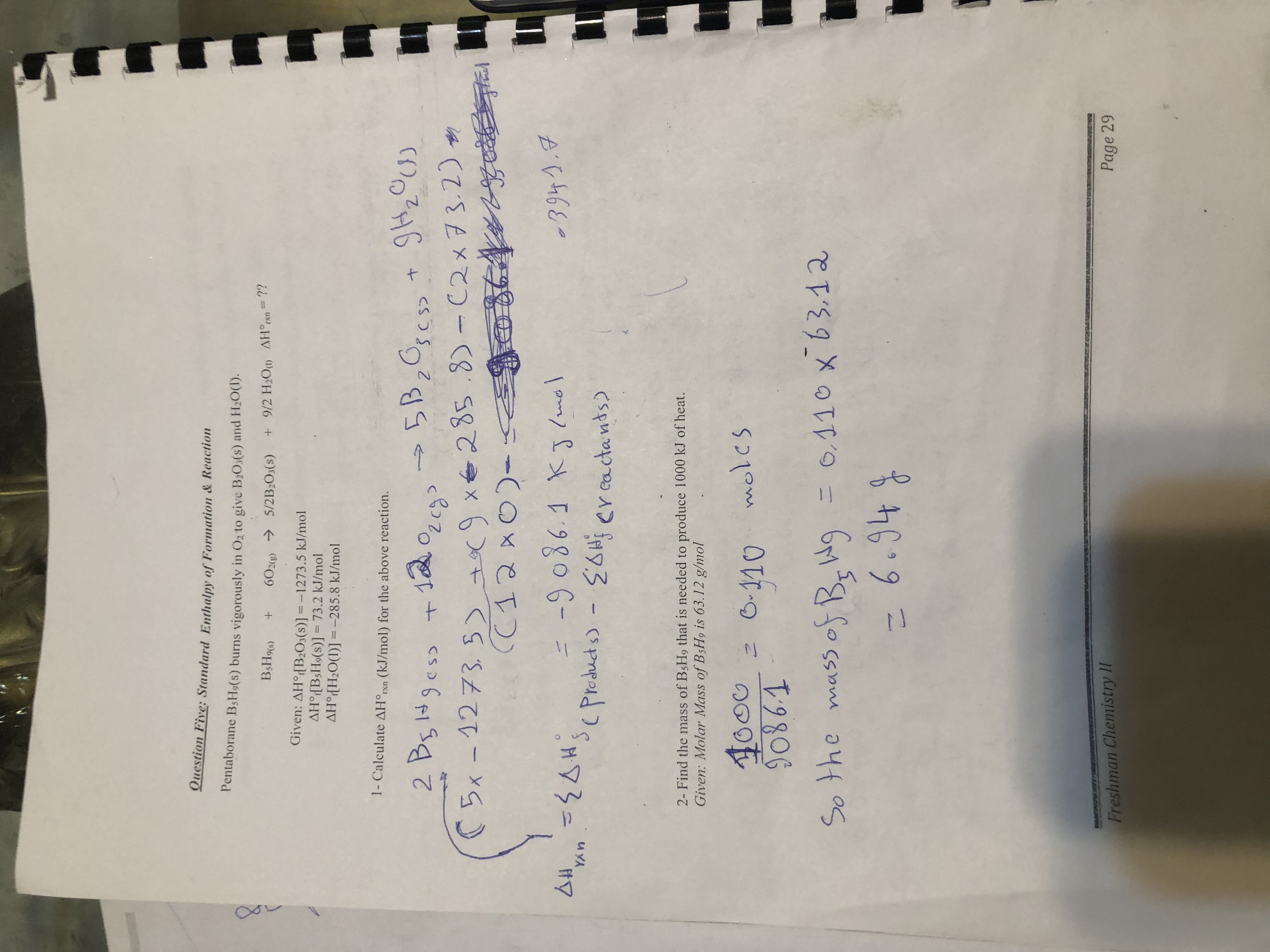# Pentaborane B5H9(s) burns vigorously in O2 to give B2O3(s) and H2O(l). B5H9(s) + 6 O2 → 5/2 B2O3(s) + 9/2 H2O(l) ∆H˚rxn =? Given: ∆H˚f [B2O3(s)] = -1273.5 kJ/mol; ∆H˚f [B5H9(s)] = 73.2 kJ/mol ∆H˚f [H2O(l)] = -285.8 kJ/mol 1. Calculate the ∆H˚rxn for the reaction above. 2. Find the mass of B5H9(s) that is needed to produce 1000 kJ of heat. Given: Molar mass of B5H9(s) is 63.12 g/mol